Alves, R. C., Mantilla, J. N. R., Bremer, C. F., and Carrasco, E. V. M. (2015). "Application of acoustic tomography and ultrasonic waves to estimate stiffness constants of Muiracatiara Brazilian wood," BioRes. 10(1), 1845-1856.

#### Abstract

Determination of the stiffness constants of Muiracatiara wood (Astronium lecointei) was performed using Fakopp 3D acoustic tomography and James V Mk II ultrasound devices. Specific gravity, moisture content, and compression perpendicular to grain tests followed standard Brazilian requirements. Statistical tests were calculated to 99% confidence intervals. Using Christoffel’s equation, equality between stiffness constants and static modulus of elasticity occurred only when using the acoustic tomography device. These results show the importance of the acoustic tomography device, not only to detect defects, but also in determining elastic constants of wood.

Application of Acoustic Tomography and Ultrasonic Waves to Estimate Stiffness Constants of Muiracatiara Brazilian Wood

Rejane C. Alves,a Judy N. R. Mantilla,b Cynara F. Bremer,c and Edgar V. M. Carrasco d*

Determination of the stiffness constants of Muiracatiara wood (Astronium lecointei) was performed using Fakopp 3D acoustic tomography and James V Mk II ultrasound devices. Specific gravity, moisture content, and compression perpendicular to grain tests followed standard Brazilian requirements. Statistical tests were calculated to 99% confidence intervals. Using Christoffel’s equation, equality between stiffness constants and static modulus of elasticity occurred only when using the acoustic tomography device. These results show the importance of the acoustic tomography device, not only to detect defects, but also in determining elastic constants of wood.

Keywords: Acoustic Tomography; Ultrasound; Astronium lecointei; Stiffness constant

Contact information: a: Department of Vegetal Sciences, Federal University of Rural Semi-Árido, Mossoró, RN, Brazil; b: Faculty of Engineering and Architecture, University FUMEC, Belo Horizonte, MG, Brazil; c: Department of Technology of Architecture and Urban Planning, Federal University of Minas Gerais, Belo Horizonte, MG, Brazil; d: Department of Structural Engineering, Federal University of Minas Gerais, Belo Horizonte, MG, Brazil; *Corresponding author: mantilla@dees.ufmg.br

INTRODUCTION

The study of wave propagation in orthotropic materials began in 1880 with the development of Christoffel’s equation (Bucur 1984). Christoffel’s work has contributed to the perception of the technique of acoustic wave propagation to estimate wood properties. Liang et al. (2008) reported that non-destructive dynamic methods (NDM) partially reflect circumstances that are not always reproducible. This leaves doubts as to the efficiency of using NDM as a way of characterizing wood and makes clear the need for more research in this area.

Most studies using the propagation of waves method are associated with ultrasonics (Bucur 2006). However, references such as Deflorio et al. (2008), Dikrallah et al. (2010), Mariño et al.(2010), Zhang et al. (2011), Li et al. (2012), and Chimenti (2014) used the principle of stress waves propagation when estimating wood properties instead. The use of acoustic waves with frequencies greater than 20 kHz is an example of these ultrasonic methods. When these waves are excited with hammer shocks, mechanical waves are emitted. Using the stress waves propagation method through acoustic tomography (AT), it is possible to determine with greater accuracy the characteristics of the timber. This is because the device provides a range of velocities instead of a single value, as the ultrasound device does. Gilbert and Smiley (2004), Deflorio et al. (2008), Wang et al. (2009), Amodei et al. (2010), Li et al. (2012), Lin et al.(2013), Alves et al. (2013) and Li et al. (2014) used the stress waves technique exclusively for obtaining a tomographic image of wood species. References Wang et al. (2002, 2004), Ross et al. (2005), and Yamazaki and Sasaki (2010) determined that this wave type may also be used to estimate wood modulus of elasticity. However, no author has used acoustic tomography (AT) to estimate wood elastic modulus yet.

Authors such as Steiger (1997), Ross et al. (1998), Lourenço et al. (2007), and Teles et al. (2011) used the dynamic elastic modulus in response to the relationship between wave propagation velocity and wood specific gravity. However, these authors made use of an equation without considering the various Poisson coefficient influences and transverse elastic modulus. Bucur (2006) and Gonçalves et al. (2011), called the product of Christoffel´s equation an elastic constant. Therefore, comparisons between the elastic constant and the dynamic elastic modulus may sometimes occur, so long as the modulus of elasticity is calculated by a static test.

Nicolotti et al. (2003) pointed out that different types of energies can provide information about the different properties of wood. Included among these energies are ultrasonic waves and their subsequent effect on the determination of elastic properties. This paper aims to use AT to estimate the elastic constants of Muiracatiara, a Brazilian wood, and compare these constants to the results determined using the ultrasonic waves propagation method.

MATERIALS AND METHODS

Propagation of Ultrasonic Waves in Wood

The wave propagation in wood can be explained using movement equations established for an anisotropic solid. These can be determined by utilizing a combination of Newton’s law and a generalized form of Hooke’s law (Bucur 1984; Carrasco and Azevedo 2003), (Eq. 1),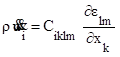(1)

where is the specific gravity, ü is acceleration, and is deformation.

Considering the definition of deformation, Eq. 2, and substituting into Eq. 1, Eq. 3 is obtained. The resulting Ciklm is a fourth degree tensor also known as an elastic constant tensor. As Ciklm is symmetrical in relation to 1, the indices in the second term within the brackets of Eq. (3) can be swapped, obtaining Eq. 4.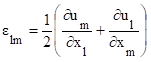(2)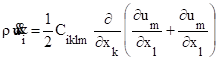(3)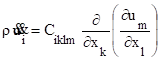(4)

Assuming that plane harmonic waves are spreading in the material, the solution of Eq. 6 is given by Eq. 5,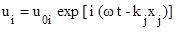(5)

wherein u0i is the amplitude of the components of the displacement vector and kj is the components of the wave vector. The value u0i can be written as u0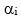, where u0 is the amplitude of the displacement andare the direction cosines of the displacement vector of the particle.

Substituting the value expressed by Eq. 5 into Eq. 4 yields Eq. 6. This equation can be written in a more homogeneous manner by making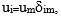where the tensor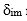is the unit tensor or, Kronecker delta (Eq. 7).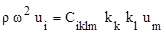(6)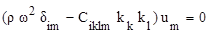(7)

Equation 7 was developed by Christoffel (Bucur 1984) and is commonly known as Christoffel’s equation. It represents a set of three homogeneous equations of first degree (linear) in u1, u2 and u3. These equations have nonzero, not trivial solutions, only if the determinant of the coefficient matrix is equal to zero (Eq. 8).

The development of this equation provides a cubic equation represented by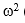(or in terms of v2). The three roots of the equation are different and generate three disparate values of propagation velocities.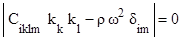(8)

In general, it is more convenient to write Eq. 7 in the form of Eq. 9, wherein tensor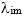, i.e. Christoffel´s tensor, is defined as Eq. 10.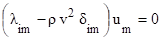or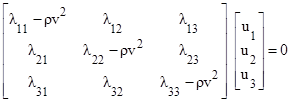(9)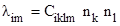(10)

In Eq. 9, v represents the phase velocity of the waves and ni (implicit in) denotes the direction cosines of the normal for the wavefronts. Thus, the new factor to be calculated is given by Eq. 11. The tensordepends on the structural symmetry and direction of the wave in the material. Thus, Eq. 11 can be rewritten in matrix form, Eq. 12.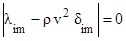(11)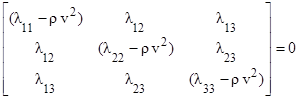(12)

It is often convenient to use matrix notation instead of tensor notation. For this, the following scheme is adopted: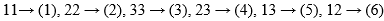. Thus, by expanding Eq. 10 and using the symmetry of the tensor Ciklm, Eq. 13 is obtained.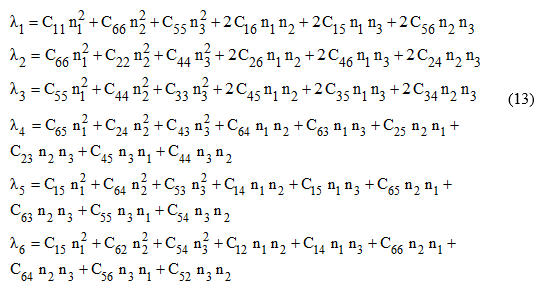A careful examination of Eq. 12 shows that the displacement vectors (eigenvectors) associated with each eigenvalue (ρv2), are mutually perpendicular. For a given direction of propagation, defined by the wave vector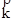, three waves propagate with displacement vectors mutually perpendicular to each other but with varying velocities. In general, these waves are not purely longitudinal or purely transverse.

However, for certain directions of propagation in a given medium material, in which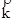is an eigenvector of, a wave is purely longitudinal and the other two are purely transverse. For a pure longitudinal wave the displacement vector of the particle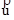is parallel to the unit vector perpendicular to the wavefronts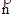. Therefore, the vector product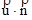is zero. On the other hand, for a pure transverse wave, the same vectors are perpendicular to each other and, consequently, the scalar productis also zero.

Christoffel has demonstrated that the direction cosinesof the displacement of the particles of the wavefronts are connected with their corresponding wave velocities, Eq. 14.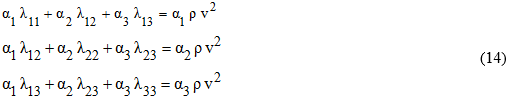This set of equations can be easily deduced from Eq. 9 by replacing um for the direction cosineswith the particledisplacement vector, Eq. 15. Thus, the known propagation velocity of the wave, propagation direction, and specific gravity of the wood allows for the determination of the elastic constants in the matrix.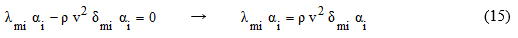The determination of elastic constants of wood can be simplified as the determination of a solid orthogonal isotropic; otherwise known as an orthotropic solid. A matrix of the elastic coefficients of an orthotropic solid is provided in Eq. 16. Thus, it is possible to distinguish three structural planes of symmetry in a wooden sample, all of which are elastic, as illustrated in Fig. 1.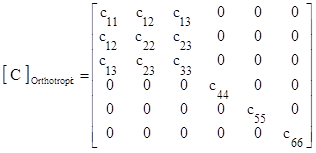(16)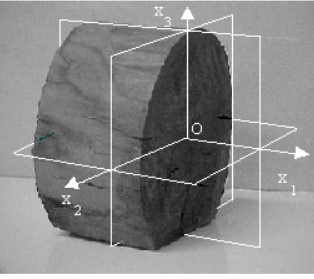Fig. 1. Planes and directions of wood symmetry

The first plane, denoted by X2OX3, whose unit normal vector is parallel to the X1 axis, is perpendicular to the direction of the wood grain. The second plane, also called the tangential plane, is defined as X1OX2. This plane’s unit normal vector follows the direction of the X3 axis and is parallel to the layers of annual growth. The third plane known as the radial plane and denoted by X1OX3, is mutually perpendicular to the other two planes. The unit normal vector of this plane is parallel to the direction of axis X2. The X2OX3, X1OX2 and X1OXparallel planes are also elastic symmetry planes. The directions of the symmetry axes X1, X2 and X3 are specifically labeled longitudinal (L), tangential (T) and radial (R).

Using the proposed method, it is possible to determine the stiffness constants (SC) in directions perpendicular to the wood grain and the average between tangential and radial fibers. After establishing the velocity of wave propagation in both AT and ultrasonic tests using Christoffel’s equation, the wood stiffness constant values were approximately estimated to be the dynamic modulus of elasticity. Equation 17 was used by Gonçalves and Bartholomew (2000), and Bucur (2006), who obtained excellent results.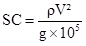(17)

In Eq. 17, the variable SC is the stiffness constant (MPa), V0 is the velocity of wave propagation (m.s-1), p is wood specific gravity, at 12% moisture content (kg.m³), and g is the acceleration of gravity 10 (m.s-2).

Material

The wood used was Muiracatiara (Astronium lecointei), which is native to the Amazon region of Brazil. For the manufacture of test pieces, cross-sections of 150×150 mm2 were cut from seven beams 2500 mm length. The samples were air-dried and subsequently cut into smaller parts. After drying, the wood pieces were stored for humidity stabilization according to Brazilian standard NBR 7190 (1997). From each wooden beam, 150 mm edge cubes were selected with an additional 14 samples taken for compression tests (tangentially and radially oriented). Two samples from each beam were also collected for moisture content and specific gravity tests. The moisture content and specific gravity of the wood samples were determined according to NBR 7190 (1997) with corrections performed using ASTM D 2395 (1998) and ISO 3130 (1975). Figure 2 shows the removal scheme of samples used in tests.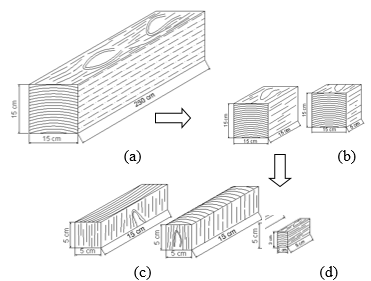Fig. 2. Removal scheme of the samples used in the tests: (a) wooden beam, (b) 15 cm edge cube and 15x15x5 cm3 piece, (c) tangential and radial directions pieces for compression tests, and (d) moisture content and specific gravity test samples

Experimental Tests

Both destructive and non-destructive tests were performed at the Advanced Research Center of Wood and New Materials in the Federal University of Minas Gerais, Brazil.

Two devices were used to determine the wave propagation velocity: the Acoustic Tomograph (AT) Fakopp 3D and James V Mk II ultrasound. The AT used 8 piezoelectric transducers while the ultrasound used two (one transmitter and one receiver). During the AT tests, 150 mm edge cubes were used, Fig. 2b. In each piece, eight sensors were attached on a horizontal plane (2 sensors per edge). The software determined a grid of 42 wave propagation velocities capable of scanning the entire surface of the wood piece (because the diagonal of the matrix is null). Through this matrix, an average velocity was computed. During the ultrasonic waves method, tests were conducted using two transducers, which were attached to cross-sections using gel. One transducer emitted a wave signal (emitter), which was captured by the other transducer (receiver).

Figure 3a displays the position of the transducers installed (high sensitivity accelerometers) and that it is ready for the beginning of the AT test. This test involved exciting each transducer through a hammer shock, which then emitted a mechanical wave. The travel time of the pulse between the excited sensor and the receivers was measured, and software calculated the velocity of each mechanical wave. After excitation of all sensors, a measuring net was obtained (Fig. 3b). The surface cross-sectional graph, or tomographic image, was determined by the values of the ratio between the velocity of the wave between two sensors and the highest velocity. The highest velocity was considered to be that of a completely healthy timber. The software transformed this information into isochromatic velocities and presented the tomographic image shown in Fig. 3c.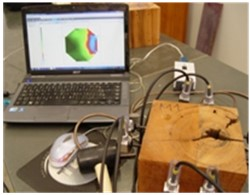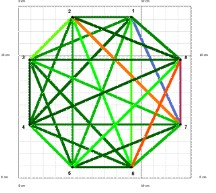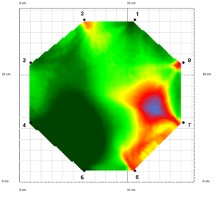(a)                                                               (b)                                                                   (c)

Fig. 3. a) Parameters used in the AT test, b) velocities of each mechanic wave, and c) tomographic image

A James V Mk II device attached to an oscilloscope for waveforms was used in tests using ultrasonic waves. The transducers had a 25 mm diameter and a 150 kHz frequency and were fixed in a spring-loaded mechanism to ensure that the pressure engagement in all tests was the same (Fig. 4).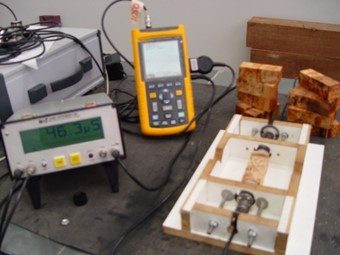Fig. 4. Ultrasonic wave tests

The normal MOE to grain is considered as the mean value between the results of the MOE’s destructive tests both in tangential and radial direction. In non-destructive tests, using ultrasound, it also used the average of the experimental results both in tangential and radial direction. In this test it used the specimens shown in Fig. 2c.

In the non-destructive testing, using acoustic tomograph, experimental results that provide the wave’s speed in normal direction to fibers, the specimens used were those shown in Fig. 2b.

RESULTS AND DISCUSSION

The average wave propagation velocity for the species Astronium lecointei was 1347 m.s-1 when the acoustic tomography device was used and 1769 m.s-1 when the ultrasonic device was used, a difference of more than 30%.

The AT test provided a velocity array (42 velocities). The highest propagation velocity, which was used as a reference, corresponded to a timber free from defects. The remaining values of the velocities matrix were determined by the device software. The values of maximum velocities were close to the values of the wave propagation velocities calculated by ultrasonic waves (Table 1). This was expected because the maximum velocity in the AT test was probably representative of sound paths for which the wood was free of defects, and velocities with ultrasound were determined in pieces totally free of defects.

Table 1 shows that the velocities of both the AT and ultrasound tests did not only depend on the specific gravity. Several authors such as Shaji et al. (2000), Wang et al. (2004), Oliveira and Sales (2005), and Bucur (2006) confirm this, further indicating that these velocities also depend on wood anatomy and chemical composition.

Table 1 shows the values of the stiffness constants, calculated by Christoffel´s equation, SC=(ρV²/g*105), Eq. 1, for AT and ultrasound tests. Performing an analysis of null difference between pairs of values (experimental modulus of elasticity versus stiffness constants) with a significance level of 99% probability, the possibility of equality between the experimental modulus of elasticity and the stiffness constants of AT tests (with confidence interval -208; 936, t-value = 2.36 and p-value = 0.056) can be concluded. For the stiffness constants of ultrasound tests, the hypothesis of equality was represented with a confidence interval of 1197; 1638, with a t-value of 22.46 and p-value of 0.000. As the confidence interval does not include zero and the p-value is very low, the difference null hypothesis is rejected, i.e. SC values are not equal.

In the graph of Fig. 5 (normalized in relation to E.M.E) it was observed that the ratio between the stiffness constants using ultrasound and the experimental modulus of elasticity ranged between 75% and 225%. As such a calibration coefficient to consider variables such as the attenuation of the wave was required. The ratio between the stiffness constants and experimental modulus of elasticity through AT test varied between -10% and 130%, with mean values close. This was expected, as 42 velocities which mapped the entire wood cross section were used in the determination of the stiffness constants through AT test.

It can also be seen in this graph that the stiffness constants through AT test values calculated with maximum velocities were close to the values of the stiffness constants through ultrasound test. This occurred because the ultrasound tests were performed on specimens free of defects, and the maximum speed in the AT test is determined in a region where the wood is free of defects.

Figure 5 shows a graph of the ratio S.C./E.M.E. (the average and maximum S.C. for both AT and US tests) and specific gravity.

Table 1. Specific Gravity Values (S.G.), Propagation Velocities (P.V.), Experimental Modulus of Elasticity (E.M.E.) and Stiffness Constants (S.C.) for AT and Ultrasound (US) Tests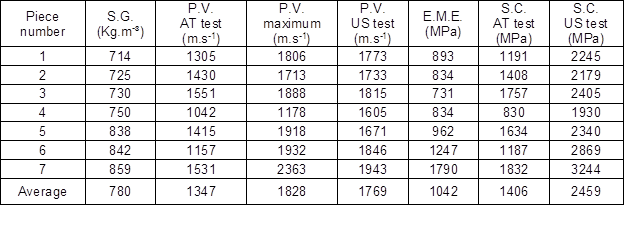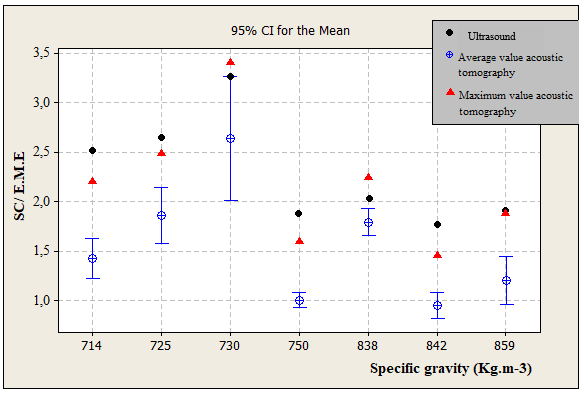Fig. 5. Experimental results

CONCLUSIONS

1. With 95% significance it can be concluded that the possibility of equality between the stiffness constants and the experimental module of elasticity occurs only for acoustic tomography tests.
2. Although the ultrasonic device is described by many authors as an efficient method for the determination of the stiffness constants through correlation equations, it proved ineffective for a direct approach as proposed in this work.
3. Stiffness constants through Acoustic Tomograph test values calculated with maximum velocities are close to the values of stiffness constants through US test.
4. Though the Acoustic Tomograph tests were carried out without the prior control of defects in the specimens, the values found were closer to the values of the static MOE than those found with the ultrasonic tests.

ACKNOWLEDGMENTS

The authors gratefully acknowledge the financial support provided by the Minas Gerais State Research Foundation (FAPEMIG).

REFERENCES CITED

Alves, R. J., Magalhaes, M. D. C., and Carrasco, E. V .M. (2013). “Determination of transverse Young’s modulus (TYM) of wood by means of an input power technique,” Construction and Building Materials 42(5), 11-21. DOI: 10.1016/j.conbuildmat.2012.12.061

Amodei, J. B., Oliveira, B. R. U., Gurgel, M. M., Carvalho, A. M., Medeiros, R. A., and Latorraca, J. V .F. (2010). “Avaliação preliminar da qualidade da madeira de Tectona grandis L. f. através da tomografia de impulso,” Floresta e Ambiente 17(2), 124-128. DOI: 10.4322/floram.2011.016

ASTM D 2395 (1998). “Standard test method for specific gravity of wood based materials,” American Society for Testing Materials, USA.

Bucur, V. (1984). “Elastic constants for wood by an ultrasonic method,” Wood Sci. Technol. 18(4), 255-265. DOI: 10.1007/BF00353361

Bucur, V. (2006). Acoustics of Wood, Springer-Verlag, Berlin, Germany.

Carrasco, E. V. M., and Azevedo Júnior, A. P. (2003). “Avaliação não-destrutiva de propriedades mecânicas de madeiras através de ultrassom – fundamentos físicos e resultados experimentais,” Cerne. 9(2), 178-191.

Chimenti, D. E. (2014). “Review of air-coupled ultrasonic materials characterization,” Ultrasonics 54(7), 1804-1816. DOI: 10.1016/j.ultras.2014.02.006

Deflorio, G., Fink, S., and Schwarze, F. W. M. R. (2008). “Detection of incipient decay in tree stems with sonic tomography after wounding and fungal inoculation,” Wood Science and Technology 42(2), 117-132. 10.1007/s00226-007-0159-0

Dikrallah, A., Kabouchi, B., Hakam, A., Brancheriau, L., Bailleres, H., Famiri, A., and Ziani, M. (2010). “Study of acoustic wave propagation through the cross section of green wood,” Comptes Rendus Mecanique 338(2), 107-112. DOI: 10.1016/j.crme.2010.02.004

Gilbert, E. A., and Smiley, E. T. (2004). “PiCUS sonic tomography for the quantification of decay in white oak (Quercus alba) and hickory (Carya spp.),” J Arboric. 30(5), 277-280.

Gonçalves, R., and Bartholomeu, A. (2000). “Avaliação do desempenho de ensaio não destrutivo em vigas de madeira de Eucalyptus citriodora Pinus elliottii,” Revista Brasileira de Engenharia Agrícola e Ambiental 4(2), 269-274. DOI: 10.1590/S1415-43662000000200023

Gonçalves, R., Trinca, A. J., and Cerri, D. G. (2011). “Comparison of elastic constants of wood determined by ultrasonic wave propagation and static compression test,” Wood and Fiber Science 43(1), 64-75.

ISO 3130 (1975). “Wood determination of moisture content for physical and mechanical tests,” International Organization for Standardization, Switzerland.

Li, G., Wang, X., Feng, H., Wiedenbeck, J., and Ross, R. J. (2014). “Analysis of wave velocity patterns in black cherry trees and its effect on internal decay detection,” Computers and Electronics in Agriculture 104, 32-39. DOI: 10.1016/j.compag.2014.03.008

Li, L., Wang, X., Wang, L., and Allison, R. B. (2012). “Acoustic tomography in relation to 2D ultrasonic velocity and hardness mappings,” Wood Sci. Technol. 46(1-3), 551-561. DOI: 10.1007/s00226-011-0426-y

Liang, S., Wang, X., Wiedenbeck, J., Cai, Z., and Fu, F. (2008). “Evaluation of acoustic tomography for tree decay detection,” Proceedings of the 15th International Symposium on Nondestructive Testing of Wood- Session 1, USA, 49-54.

Lin, C. J., Chung, C. H., Wu, M. L. and Cho, C. L. (2013). “Detection of Phellinus noxius decay in Sterculia foetida tree,” Journal of Tropical Forest Science 25(4), 487-496.

Lourenço, P. B., Feio, A. O., and Machado, J. S. (2007). “Chestnut wood in compression perpendicular to the grain: Non-destructive correlations for test results in new and old wood,” Construction and Building Materials 21(8), 1617-1627. DOI: 10.1016/j.conbuildmat.2006.07.011

Mariño, R. A., Fernandez, M. E., Fernandez-Rodríguez, C., and Méndez, M. (2010). “Detection of pith location in chestnut lumber (Castanea sativa Mill.) by means of acoustic tomography and longitudinal stress-wave velocity,” Eur. J. Wood Prod. 68(2), 197-206. DOI: 10.1007/s00107-009-0366-5

NBR 7190 (1997). Projeto de Estruturas de Madeira, Associação Brasileira de Normas Técnicas, Rio de Janeiro, Brazil.

Nicolotti, G., Socco, L.V., Martinis, R., Godio, A., and Sambuelli, L. (2003). “Application and comparison of three tomographic techniques for detection of decay in trees,” Journal of Arboriculture 29(2), 66-78.

Oliveira, F. G. R., and Sales, A. (2005). “Efeito da densidade e do teor de umidade na velocidade ultrasônica da madeira,” Minerva 2(1), 25-31.

Ross, R. J., Brashaw, B. K., and Pellerin, R. F. (1998). “Nondestructive evaluation of wood,” Forest Products Journal 48(1), 14-19.

Ross, R. J., Zerbe, J. I., Wang, X. P., Green, D. W., and Pellerin, R. F. (2005). “Stress wave nondestructive evaluation of Douglas-fir peeler cores,” Forest Products Journal 55(3), 90-94.

Shaji, T., Somayaji, S., and Mathews, M. S. (2000). “Ultrasonic pulse velocity technique for inspection and evaluation of timber,” Journal of Materials in Civil Engineering 12(2), 180-185. DOI: 10.1061/(ASCE)0899-1561(2000)12:2(180)

Steiger, R. (1997). “Sortierung von Rund-und Schnittholz mittels Ultraschall,” Holzforschung und Holzverwertung 49(2), 28-35.

Teles, R. F., Del Menezzi, C. S., Souza, F., and Souza, M. R. (2011). “Nondestructive evaluation of a tropical hardwood: Interrelationship between methods and physical-acoustical variables,” Ciência da Madeira 2(1), 1-14. DOI: 10.12953/2177-6830.v02n01a01

Wang, X. P., Ross, R. J., Mattson, J. A., Erickson, J. R., Forsman, J. W., Geske, E. A., and Wehr, M. A. (2002). “Nondestructive evaluation techniques for assessing modulus of elasticity and stiffness of small-diameter logs,” Forest Products Journal 52(2), 79-85.

Wang, X., Ross, R. J., Green, D. W., Brashan, B. K., Englund, K. and Wolcott, M. (2004). “Stress wave sorting of red maple logs for structural quality,” Wood Sci. Technol. 37(6), 531-537. DOI: 10.1007/s00226-003-0202-8

Wang, X., Wiedenbeck, J., and Liang, S. (2009). “Acoustic tomography for decay detection in black cherry trees,” Wood Fiber Sci. 41(2), 127-137.

Yamazaki, M., and Sasaki, Y. (2010). “Determining Young’s modulus of timber on the basis of a strength database and stress wave propagation velocity I: An estimation method for Young’s modulus employing Monte Carlo simulation,” J. Wood Sci. 56(4), 269-275. DOI: 10.1007/s10086-010-1108-3

Zhang, H., Wang, X. and Su, J. (2011). “Experimental investigation of stress wave propagation in standing trees,” Holzforschung 65(5), 743-748. DOI: 10.1515/hf.2011.059

Article submitted: September 16, 2014; Peer review completed: December 12, 2014; Revised version received and accepted: January 29, 2015; Published: January 30, 2015.